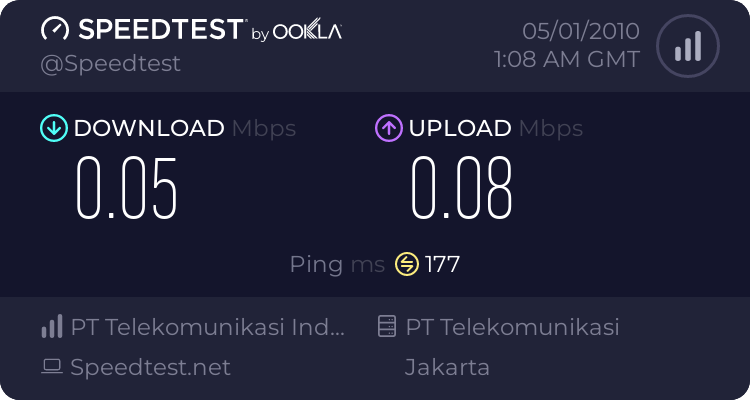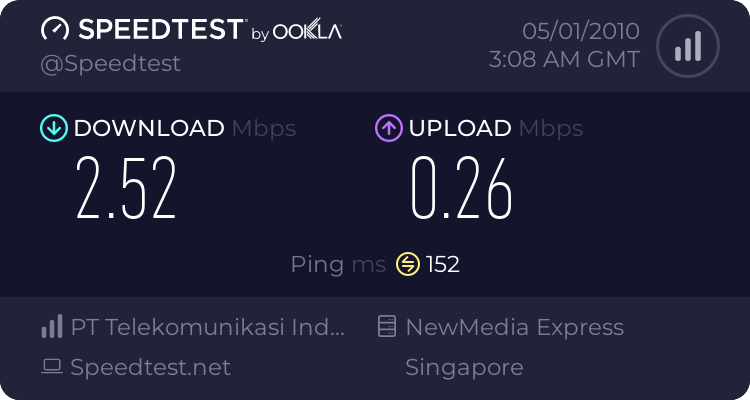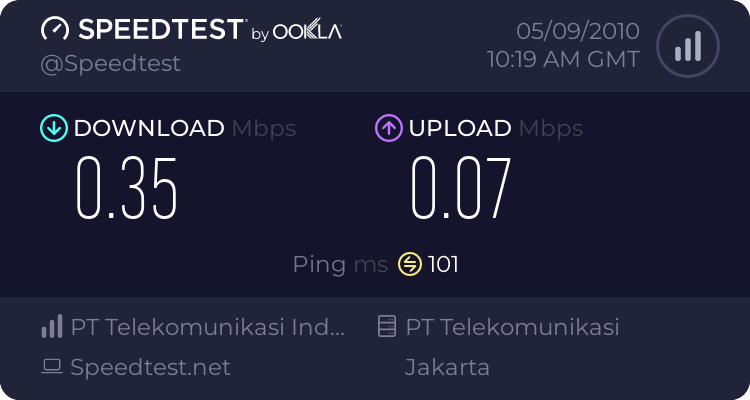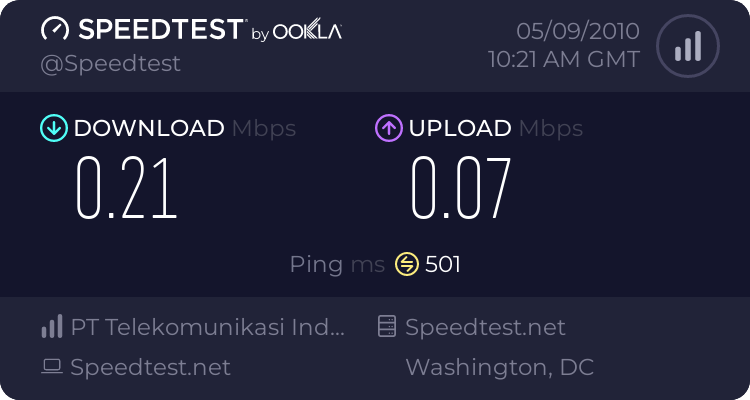ISP
Home > LOEKELOE > COMPUTER STUFF > Internet Service & Networking > ISP > #ABSEN# Speedy yang IX-nya ancur hari ini...
Total Views: 2262 Share :FacebookTwitterGoogle+
Page 4 of 6 |  < 1 2 3 4 5 6 >

xirenia - 30/04/2010 06:21 PM
#61

[HTML]Reply from 69.63.181.11: bytes=32 time=358ms TTL=239
Request timed out.
Reply from 69.63.181.11: bytes=32 time=337ms TTL=241
Reply from 69.63.181.11: bytes=32 time=357ms TTL=239
Reply from 69.63.181.11: bytes=32 time=336ms TTL=239
Reply from 69.63.181.11: bytes=32 time=360ms TTL=241
Request timed out.
Request timed out.
Reply from 69.63.181.11: bytes=32 time=754ms TTL=241
Reply from 69.63.181.11: bytes=32 time=720ms TTL=239
Reply from 69.63.181.11: bytes=32 time=868ms TTL=239
Request timed out.
Reply from 69.63.181.11: bytes=32 time=505ms TTL=239
Reply from 69.63.181.11: bytes=32 time=352ms TTL=239
Reply from 69.63.181.11: bytes=32 time=359ms TTL=239
Reply from 69.63.181.11: bytes=32 time=355ms TTL=241
Reply from 69.63.181.11: bytes=32 time=338ms TTL=241
Reply from 69.63.181.11: bytes=32 time=356ms TTL=239
Request timed out.
Request timed out.
Request timed out.
Reply from 69.63.181.11: bytes=32 time=338ms TTL=241
Reply from 69.63.181.11: bytes=32 time=364ms TTL=239
Reply from 69.63.181.11: bytes=32 time=351ms TTL=239[/HTML]

Keren yaa?
rudte4 - 30/04/2010 06:38 PM
#62

Quote:
Original Posted By xirenia
[HTML]Reply from 69.63.181.11: bytes=32 time=358ms TTL=239
Request timed out.
Reply from 69.63.181.11: bytes=32 time=337ms TTL=241
Reply from 69.63.181.11: bytes=32 time=357ms TTL=239
Reply from 69.63.181.11: bytes=32 time=336ms TTL=239
Reply from 69.63.181.11: bytes=32 time=360ms TTL=241
Request timed out.
Request timed out.
Reply from 69.63.181.11: bytes=32 time=754ms TTL=241
Reply from 69.63.181.11: bytes=32 time=720ms TTL=239
Reply from 69.63.181.11: bytes=32 time=868ms TTL=239
Request timed out.
Reply from 69.63.181.11: bytes=32 time=505ms TTL=239
Reply from 69.63.181.11: bytes=32 time=352ms TTL=239
Reply from 69.63.181.11: bytes=32 time=359ms TTL=239
Reply from 69.63.181.11: bytes=32 time=355ms TTL=241
Reply from 69.63.181.11: bytes=32 time=338ms TTL=241
Reply from 69.63.181.11: bytes=32 time=356ms TTL=239
Request timed out.
Request timed out.
Request timed out.
Reply from 69.63.181.11: bytes=32 time=338ms TTL=241
Reply from 69.63.181.11: bytes=32 time=364ms TTL=239
Reply from 69.63.181.11: bytes=32 time=351ms TTL=239[/HTML]

Keren yaa?

ip 222.124

[HTML]Reply from 69.63.181.12: bytes=32 time=299ms TTL=243
Reply from 69.63.181.12: bytes=32 time=297ms TTL=243
Reply from 69.63.181.12: bytes=32 time=295ms TTL=243
Reply from 69.63.181.12: bytes=32 time=300ms TTL=243
Reply from 69.63.181.12: bytes=32 time=298ms TTL=243
Reply from 69.63.181.12: bytes=32 time=300ms TTL=243
Reply from 69.63.181.12: bytes=32 time=297ms TTL=243
Reply from 69.63.181.12: bytes=32 time=297ms TTL=243
Reply from 69.63.181.12: bytes=32 time=300ms TTL=243
Reply from 69.63.181.12: bytes=32 time=298ms TTL=243
Reply from 69.63.181.12: bytes=32 time=297ms TTL=243
Reply from 69.63.181.12: bytes=32 time=295ms TTL=243
Reply from 69.63.181.12: bytes=32 time=297ms TTL=243
Reply from 69.63.181.12: bytes=32 time=298ms TTL=243
Reply from 69.63.181.12: bytes=32 time=295ms TTL=243
Reply from 69.63.181.12: bytes=32 time=295ms TTL=243
Reply from 69.63.181.12: bytes=32 time=296ms TTL=243
Reply from 69.63.181.12: bytes=32 time=300ms TTL=243
Reply from 69.63.181.12: bytes=32 time=297ms TTL=243
Reply from 69.63.181.12: bytes=32 time=297ms TTL=243
Reply from 69.63.181.12: bytes=32 time=300ms TTL=243
Reply from 69.63.181.12: bytes=32 time=297ms TTL=243
Reply from 69.63.181.12: bytes=32 time=298ms TTL=243
Reply from 69.63.181.12: bytes=32 time=297ms TTL=243[/HTML]
Zangetsu™ - 30/04/2010 10:17 PM
#63

Quote:
lokasinya temennya sama ga?
kan posisi menentukan prestasi ...beda lokasi sih gantapi sebel juga sih paket 50 jam ngalahin yg eksekutipQuote:
Original Posted By xirenia
[HTML]Reply from 69.63.181.11: bytes=32 time=358ms TTL=239
Request timed out.
Reply from 69.63.181.11: bytes=32 time=337ms TTL=241
Reply from 69.63.181.11: bytes=32 time=357ms TTL=239
Reply from 69.63.181.11: bytes=32 time=336ms TTL=239
Reply from 69.63.181.11: bytes=32 time=360ms TTL=241
Request timed out.
Request timed out.
Reply from 69.63.181.11: bytes=32 time=754ms TTL=241
Reply from 69.63.181.11: bytes=32 time=720ms TTL=239
Reply from 69.63.181.11: bytes=32 time=868ms TTL=239
Request timed out.
Reply from 69.63.181.11: bytes=32 time=505ms TTL=239
Reply from 69.63.181.11: bytes=32 time=352ms TTL=239
Reply from 69.63.181.11: bytes=32 time=359ms TTL=239
Reply from 69.63.181.11: bytes=32 time=355ms TTL=241
Reply from 69.63.181.11: bytes=32 time=338ms TTL=241
Reply from 69.63.181.11: bytes=32 time=356ms TTL=239
Request timed out.
Request timed out.
Request timed out.
Reply from 69.63.181.11: bytes=32 time=338ms TTL=241
Reply from 69.63.181.11: bytes=32 time=364ms TTL=239
Reply from 69.63.181.11: bytes=32 time=351ms TTL=239[/HTML]

Keren yaa?

Spoiler for ping

Reply from 69.63.181.11: bytes=32 time=328ms TTL=244
Reply from 69.63.181.11: bytes=32 time=437ms TTL=244
Reply from 69.63.181.11: bytes=32 time=273ms TTL=244
Reply from 69.63.181.11: bytes=32 time=251ms TTL=244
Reply from 69.63.181.11: bytes=32 time=245ms TTL=244
Reply from 69.63.181.11: bytes=32 time=243ms TTL=244
Reply from 69.63.181.11: bytes=32 time=432ms TTL=244
Reply from 69.63.181.11: bytes=32 time=351ms TTL=244
Reply from 69.63.181.11: bytes=32 time=339ms TTL=244
Reply from 69.63.181.11: bytes=32 time=252ms TTL=244
Reply from 69.63.181.11: bytes=32 time=334ms TTL=244
Reply from 69.63.181.11: bytes=32 time=246ms TTL=244
Reply from 69.63.181.11: bytes=32 time=244ms TTL=244
Reply from 69.63.181.11: bytes=32 time=359ms TTL=244
Request timed out.
Reply from 69.63.181.11: bytes=32 time=316ms TTL=244
Reply from 69.63.181.11: bytes=32 time=785ms TTL=244
Request timed out.
Reply from 69.63.181.11: bytes=32 time=359ms TTL=244
Reply from 69.63.181.11: bytes=32 time=252ms TTL=244
Reply from 69.63.181.11: bytes=32 time=912ms TTL=244
Reply from 69.63.181.11: bytes=32 time=524ms TTL=244
Reply from 69.63.181.11: bytes=32 time=441ms TTL=244
Reply from 69.63.181.11: bytes=32 time=257ms TTL=244
Reply from 69.63.181.11: bytes=32 time=253ms TTL=244
Request timed out.
Reply from 69.63.181.11: bytes=32 time=238ms TTL=244
Reply from 69.63.181.11: bytes=32 time=501ms TTL=244
Reply from 69.63.181.11: bytes=32 time=493ms TTL=244
Reply from 69.63.181.11: bytes=32 time=300ms TTL=244
Reply from 69.63.181.11: bytes=32 time=383ms TTL=244
Reply from 69.63.181.11: bytes=32 time=774ms TTL=244
Reply from 69.63.181.11: bytes=32 time=247ms TTL=244
Request timed out.
Request timed out.
Reply from 69.63.181.11: bytes=32 time=372ms TTL=244
Reply from 69.63.181.11: bytes=32 time=243ms TTL=244
Reply from 69.63.181.11: bytes=32 time=563ms TTL=244
Reply from 69.63.181.11: bytes=32 time=576ms TTL=244
Reply from 69.63.181.11: bytes=32 time=424ms TTL=244
Reply from 69.63.181.11: bytes=32 time=401ms TTL=244
Reply from 69.63.181.11: bytes=32 time=424ms TTL=244
Reply from 69.63.181.11: bytes=32 time=380ms TTL=244
Reply from 69.63.181.11: bytes=32 time=240ms TTL=244

hampir sama pingnya, speed IX tetep lelet-mamayukero - 30/04/2010 11:52 PM
#64

speedy kurang ajiar ane ga bisa download padahal sudah beli rapid premium 1 tahun..... dasar bekicotMerky - 01/05/2010 03:48 AM
#65

parah neh speedy.... game facebook ngelag semua.....................
Toby - 01/05/2010 09:06 AM
#66

Gw daerah Bandung lambret jg bos paket family, bener2 dah, nelpon 147 dibilang lambet gara2 turun 128 kbps, ini dah tanggal 1 lambetnya sama2 ajaini speed test aja lambetnya minta ampundidjital - 01/05/2010 11:09 AM
#67

Update May 1 2010

Pinging a2047.w7.akamai.net [61.213.183.105] with 32 bytes of data:

Reply from 61.213.183.105: bytes=32 time=157ms TTL=51
Reply from 61.213.183.105: bytes=32 time=154ms TTL=51
Reply from 61.213.183.105: bytes=32 time=155ms TTL=51
Reply from 61.213.183.105: bytes=32 time=155ms TTL=51
Reply from 61.213.183.105: bytes=32 time=157ms TTL=51
Reply from 61.213.183.105: bytes=32 time=157ms TTL=51
Reply from 61.213.183.105: bytes=32 time=154ms TTL=51
Reply from 61.213.183.105: bytes=32 time=155ms TTL=51
Reply from 61.213.183.105: bytes=32 time=163ms TTL=51
Reply from 61.213.183.105: bytes=32 time=159ms TTL=51
Reply from 61.213.183.105: bytes=32 time=162ms TTL=51
Reply from 61.213.183.105: bytes=32 time=153ms TTL=51
Reply from 61.213.183.105: bytes=32 time=156ms TTL=51
Reply from 61.213.183.105: bytes=32 time=154ms TTL=51
Reply from 61.213.183.105: bytes=32 time=153ms TTL=51
Reply from 61.213.183.105: bytes=32 time=258ms TTL=51
Reply from 61.213.183.105: bytes=32 time=154ms TTL=51
Reply from 61.213.183.105: bytes=32 time=154ms TTL=51
Reply from 61.213.183.105: bytes=32 time=164ms TTL=51
Reply from 61.213.183.105: bytes=32 time=155ms TTL=51
Reply from 61.213.183.105: bytes=32 time=156ms TTL=51
Reply from 61.213.183.105: bytes=32 time=164ms TTL=51
Reply from 61.213.183.105: bytes=32 time=156ms TTL=51
Reply from 61.213.183.105: bytes=32 time=155ms TTL=51
Reply from 61.213.183.105: bytes=32 time=154ms TTL=51
Reply from 61.213.183.105: bytes=32 time=167ms TTL=51
Reply from 61.213.183.105: bytes=32 time=154ms TTL=51
Reply from 61.213.183.105: bytes=32 time=153ms TTL=51
Reply from 61.213.183.105: bytes=32 time=158ms TTL=51

Ping statistics for 61.213.183.105:
Packets: Sent = 29, Received = 29, Lost = 0 (0% loss),
Approximate round trip times in milli-seconds:
Minimum = 153ms, Maximum = 258ms, Average = 160ms[/CODE]

Akhirnya dapet route terbaik. Jogja lagi bolong....

Speedtest
Spoiler for speedtestTop Markotop. Nyus marginyus... Ada gunanya juga jadi tukang komplen. Diperhatiin.. hihi!
HAVOCprojects - 09/05/2010 06:23 PM
#68

Speedy paket 384kbps (famili >- socialia), lokasi di Bandung. Ini hasilnya:

LOKALINTERNASIONAL (ke ibukotanya amrik)FortressMU - 09/05/2010 09:07 PM
#69

^

inter emang parah saat inilokal nya maknyus :thumbup
ackotrooper - 10/05/2010 12:43 PM
#70

Quote:
Original Posted By Merky
parah neh speedy.... game facebook ngelag semua.....................

sama bro, games flash di facebook tmpt gw jg parah banget, tapi aneh nya kalo browsing sama donlod situs luar bs full load.
agoenkz - 10/05/2010 01:18 PM
#71

buest link international mati semua lagi neeh T_T!
heki - 10/05/2010 01:19 PM
#72

bener om, ix nya mati.

cibe emang speedy

gw di bandung
LostHeaven - 10/05/2010 01:21 PM
#73

kampret tiba2 ga bisa buka web luaragoenkz - 10/05/2010 01:23 PM
#74

yoii gw jg dibandung, barusan aja mati link ke ix nya, klo donlotnya udah dr pagi cuman dpt 10KB/s pdhl paket game 1MBps >.
Puxxa - 10/05/2010 05:33 PM
#75

mantap speedytexhnolyze - 10/05/2010 05:46 PM
#76nyesel dah modem m2 ane d pinjemin ke temen..
yuthz - 10/05/2010 05:50 PM
#77

anjiiing gw baru aja pasang speedy eh udah kacrut lagi gak bisa buka link luar tae tae tae
GabriJack - 10/05/2010 05:57 PM
#78

hhh ... pdhal bbrp jam yll msh kna ...
#79

Quote:
Original Posted By texhnolyzepenyesalan selalu datang terlambat